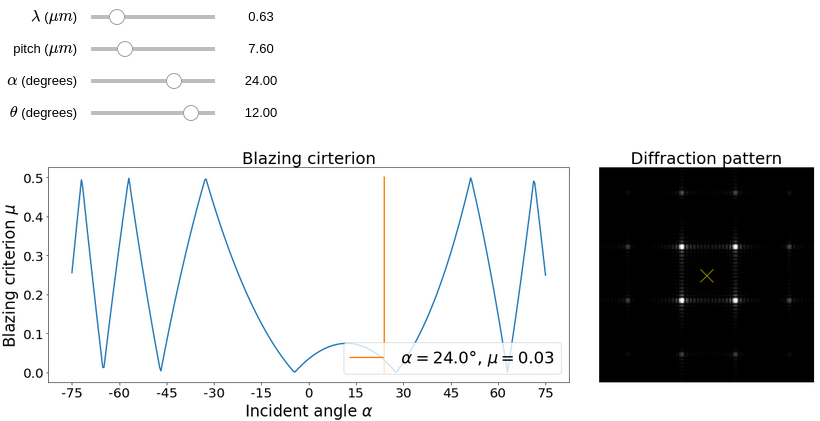Created by sebastien.popoff on 23/06/2020

## DMD diffraction toolI presented in this tutorial the diffraction effects occurring in a DMD setup. It corresponds to a blazed grating effect and depends on the wavelength $$\lambda$$, the pixel pitch $$d$$, the incident angle $$\alpha$$, and the angle of the micro-mirrors $$\theta$$. Here is a simple app (see source code on Github) to calculate the criterion for optimal diffraction efficiency and the aspect of the diffraction pattern in the far-field when illuminating the DMD with a plane wave of incident angle $$\alpha$$ with respect to the normal of the surface.